### Contrasts

You can use the CONTRAST statement to perform custom hypothesis tests. If the hypothesis is testable in the univariate case, the Wald F statistic for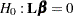is computed as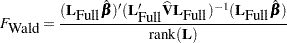whereis the contrast vector or matrix you specify,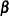is the vector of regression parameters,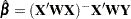,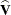is the estimated covariance matrix of, rank() is the rank of, and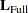is a matrix such that

•has the same number of columns as•has full row rank

• the rank ofequals the rank of thematrix

• all rows ofare estimable functions

• the Wald F statistic computed using thematrix is equivalent to the Wald F statistic computed by using thematrix with any row deleted that is a linear combination of previous rows

Ifis a full-rank matrix and all rows ofare estimable functions, thenis the same as. It is possible thatmatrix cannot be constructed for contrasts in a CONTRAST statement, in which case the contrasts are not testable.